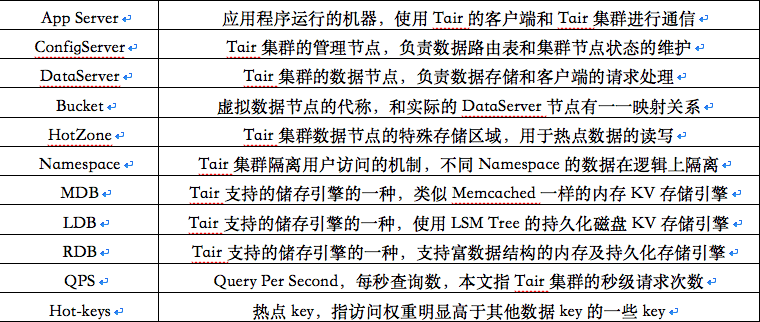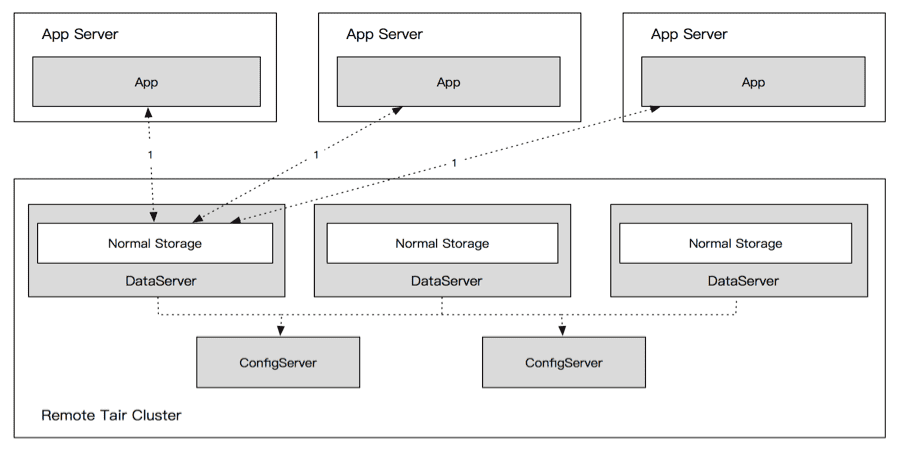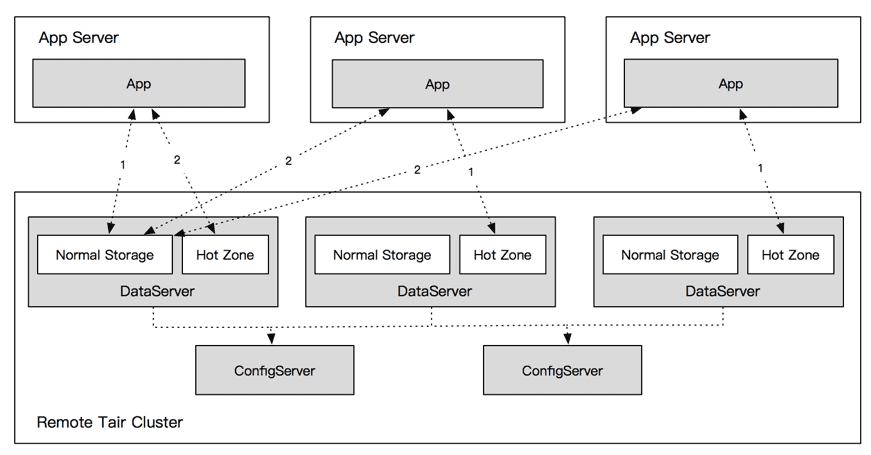# 2017双11技术揭秘—分布式缓存服务Tair的热点数据散列机制

2017/12/29 16:07## 1 问题背景

Tair是阿里巴巴集团自研的弹性缓存/存储平台，在内部有着大量的部署和使用。Tair的核心组件是一个高性能、可扩展、高可靠的NoSQL存储系统。目前支持MDB、LDB、RDB等存储引擎。其中MDB是类似Memcached的内存存储引擎，LDB是使用LSM Tree的持久化磁盘KV存储引擎，RDB是支持Queue、Set、Maps等数据结构的内存及持久化存储引擎。

Tair的数据分片和路由算法采用了Amazon于2007年提出的一种改进的一致性哈希算法。该算法将整个哈希空间分为若干等大小的Q份数据分区（也称为虚拟节点，Q>>N，N为缓存节点数），每个缓存节点依据其处理能力分配不同数量的数据分区。客户端请求的数据Key值经哈希函数映射至哈希环上的位置记为token，token值再次被哈希映射为某一分区标识。得到分区标识后，客户端从分区服务器映射表中查询存放该数据分区的缓存节点后进行数据访问。使用该算法对相同数据Key进行计算，其必然会被映射到固定的DataServer上，如图：## 2 解决方案

### 2.2 读热点方案

#### 2.2.1 服务端设计#### 2.2.2客户端设计

2.2.2.1 客户端逻辑

2.2.2.2 散列比和QPS偏差的关系

A=(C+H)/N

P_c=(C/N)/A=(C/N)/((C+H)/N)=C/(C+H) ，当 H=0 时，P_c=1

P_h=(C/N+H)/A=(C/N+H)/((C+H)/N)=(C+HN)/(C+H) ，当 H=0 时，P_h=1

P_(h')=(C/N+H/M)/A=(C/N+H/M)/((C+H)/N)=(CM+HN)/(M(C+H))

P_(h')=(CM+HN)/(M(C+H))=(CKN+HN)/(KN(C+H))=(CK+H)/(K(C+H))，当 K=1 时， P_(h')=1

### 2.3 写热点方案

2.3.1.1 处理方式

#### 2.3.3 性能指标

LDB集群实际压测效果为单Key合并能做到单Key百万的QPS（1ms合并，不限制合并次数），线上实际集群为了尽可能保证实时性，均采用了最大0.1ms以及单次最大合并次数为100次的限制。这样单Key在引擎层的最大落盘QPS就能控制在10000以下（而合并的QPS则取决于应用的访问频率）。Tair服务端的包处理是完全异步化的，进行热点请求的合并操作并不阻塞对其他请求的处理。唯一的影响就是增大客户端对热点key的写请求的RT. 按照现在的配置，最坏情况下，客户端的热点key的写操作会增大0.1ms，这个影响是微乎其微的。0 评论
0 收藏
0